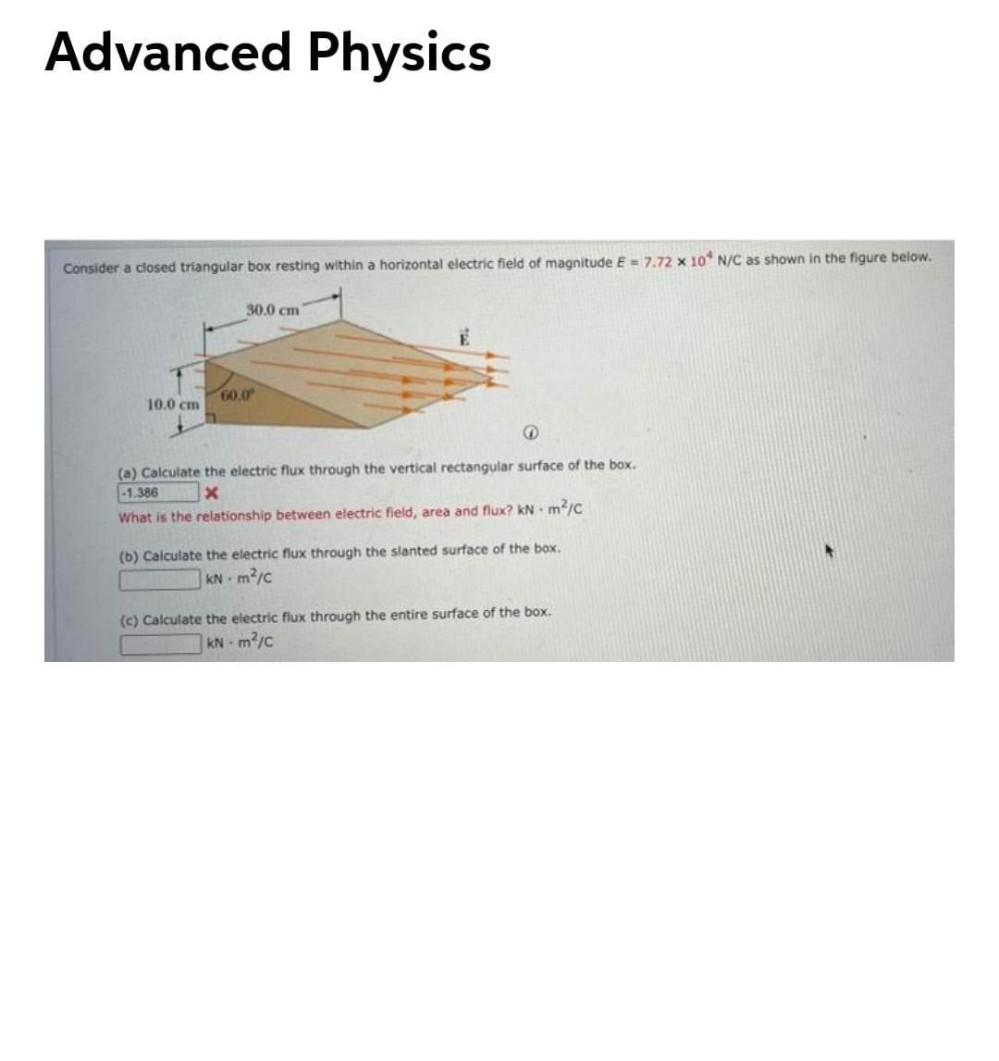Question:

# Advanced Physics Consider a closed triangular box resting within a horizontal electric field of magnitude E = 7.72 x 10' N/C asAdvanced Physics Consider a closed triangular box resting within a horizontal electric field of magnitude E = 7.72 x 10' N/C as shown in the figure below. 30.0 cm 60.0 10.0 cm -1.388 (a) Calculate the electric flux through the vertical rectangular surface of the box. x What is the relationship between electric field, area and flux? kNm²/C (b) Calculate the electric flux through the slanted surface of the box KN mic (c) Calculate the electric flux through the entire surface of the box. KN - m?c